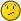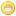[MSE] Help on a special kind of functional equation.MphLeeLong Time FellowPosts: 374 Threads: 30 Joined: May 2013 06/10/2021, 12:21 PM Hi, I know this is not directly related to tetration. It is related to iteration theory and solving functional equations. https://math.stackexchange.com/questions...alpha-beta The question is the following $f(g,\beta\alpha,x+y)=f(g^\alpha,\beta,x)f(g,\alpha,y)$ Mind that here x,y can be thought to be numbers. But the crucial part is that $\alpha$, $\beta$ and $g$ are intended to be matrices, formal powerseries or functions (non commutative objects in general) under composition. I ask also here because with JmsNxn deep familiarity with iterated composition (in the question i rendered the Omega notation as a product to not scare away normies) I could get some knowledge. MSE MphLee Mother Law $(\sigma+1)0=\sigma (\sigma+1)$ S Law $\bigcirc_f^{\lambda}\square_f^{\lambda^+}(g)=\square_g^{\lambda}\bigcirc_g^{\lambda^+}(f)$JmsNxnUltimate FellowPosts: 1,214 Threads: 126 Joined: Dec 2010 06/11/2021, 02:10 AM (This post was last modified: 06/11/2021, 03:50 AM by JmsNxn.) Is this some kind of semi-direct product? Jesus; that would be cool... Let me think on this... I'll see if I can think of anything. Is $g^\alpha$ really supposed to be an element of $\text{Aut}$; rather than necessarily a conjugation? ........ Update: So I'm not certain I can answer this; but, did you understand when I introduced the congruent integral (if you read the paper)? This is a different form of the compositional integral that is in a "modded out space". I can reduce (I think); your question into the abelian case; but I need to know how much of the congruent integral I should explain. Also; what the solutions $f$ are referred to as is homormorphisms of the semi-direct products between two groups. In this case you are taking an inner automorphism $g^{\alpha}\in \text{InnAut}(G) \subset \text{Aut}(G)$. Then you are constructing what is typically written, $G \propto_{g^\alpha} N\\$ And you are looking for $f : G \propto_{g^\alpha} N \to G$ There's a word for this; I can't remember it exactly. Also, this forum doesn't have the best latex implementation; so $\propto$ should actually be the symbol $\rtimes$; which is more angular.  It should look more like this..... I'll explain this better tomorrow. Long night; but it's at least SOMETHING like this. Nonetheless; your answer lies in semi-direct products.JmsNxnUltimate FellowPosts: 1,214 Threads: 126 Joined: Dec 2010 06/13/2021, 08:38 AM (This post was last modified: 06/13/2021, 09:42 AM by JmsNxn.) I finally can answer your question. I was a little confused about the details before; but it's definitely a semi-direct product. Let's first of all, ignore the function $f$. Let's write a product, $(xy,\beta \alpha) = (\varphi(\alpha)(x),\beta)(y, \alpha)\\ x,y \in N\,\,\alpha,\beta \in G\\$ And call this group, $N \propto_\varphi G\\$ Where $\varphi(\alpha) : G \to \text{Aut}(N)$. Where $\text{Aut}(N)$ is the group of isomorphisms of $N$. Now; there are many choices of $\varphi(\alpha)$ (something to do with Euler's phi function will be involved in the estimate of how many). Now when you write $g^{\alpha} = \alpha g \alpha^{-1} \in \text{Aut}(G)$, you are choosing an isomorphism of $\text{Aut}(G) \to \text{Aut}(N)$; let's call this $\psi : \text{Aut}(G) \to \text{Aut}(N)$. We can do this because we're only going to care about $f$ in the final result. And this is just considering $f(g,\alpha,x)$ at an implicit level in the preimage and considering it equivalent. Now, here is where I wasn't making any sense before. You wrote your equation backwards from the usual semi-direct product. The right way I should've written; which I apologize for saying. Is that it's, $N \propto_{\psi(g)} G = N \propto_{\varphi} G\\$ You are now looking for projections, $f(N \propto_{\psi(g)} G) \to G\\$ I fucking knew it was semi-direct products! Took me a while to think about it though... Regards, James. I hope this helps. Long story short; you have a lot of group theory at your disposal, Mphlee. May I recommend dummit & foote.MphLeeLong Time FellowPosts: 374 Threads: 30 Joined: May 2013 06/14/2021, 01:55 PM Yes! That's very promising. I'll have to work hard on this. Thank you so much! I believe that it is the solution: I just need to rephrase it, play with it a bit and prove it with my hands. This problem popped out at the intersection between the abstract Jabotinky foundation and superfunction-spaces. Can't wait to find time to work on the details. Thank you again.... nobody there on MSE even had the kindness to drop a single keyword. For group theorist this should be the abc... for sure my question is not well written, my English su*x, but I don't feel is a bad question. MSE MphLee Mother Law $(\sigma+1)0=\sigma (\sigma+1)$ S Law $\bigcirc_f^{\lambda}\square_f^{\lambda^+}(g)=\square_g^{\lambda}\bigcirc_g^{\lambda^+}(f)$JmsNxnUltimate FellowPosts: 1,214 Threads: 126 Joined: Dec 2010 06/14/2021, 09:52 PM I think your question was written very well. It was probably more suited to Mathoverflow though; stack exchange tends to be mostly for undergraduate homework problems. But even then, the community can be a tad abrasive; and they always poke holes in technicalities while ignoring the larger picture. I deleted my stack exchange accounts a long time ago; bored by how little they actually help. Sometimes, you have to be left to your own wits. I suspect though, no one saw that it was a semi-direct product... just a weird one. Only reason I saw it was because I was thinking about bullet notation. And I was initially trying to give an example of your function. Regards, James « Next Oldest | Next Newest »

 Possibly Related Threads… Thread Author Replies Views Last Post double functional equation , continuum sum and analytic continuation tommy1729 6 450 03/05/2023, 12:36 AM Last Post: tommy1729 [special] binary partition zeta function tommy1729 1 182 02/27/2023, 01:23 PM Last Post: tommy1729 [MSE] iterated sin using Besselfunction 1st kind Gottfried 7 769 12/18/2022, 02:06 PM Last Post: Gottfried tommy's displacement equation tommy1729 1 446 09/16/2022, 12:24 PM Last Post: tommy1729 Functional power Xorter 3 4,097 07/11/2022, 06:03 AM Last Post: CatullusFunctional Square Root Catullus 24 3,176 07/01/2022, 09:17 PM Last Post: tommy1729 A special equation : f^[t](x) = f(t x)/t tommy1729 6 1,166 06/17/2022, 12:16 PM Last Post: tommy1729 Modding out functional relationships; An introduction to congruent integration. JmsNxn 3 1,983 06/23/2021, 07:07 AM Last Post: JmsNxn Moving between Abel's and Schroeder's Functional Equations Daniel 1 4,149 01/16/2020, 10:08 PM Last Post: sheldonisonLogical tetration equation Xorter 0 3,641 02/01/2018, 10:29 PM Last Post: Xorter

Users browsing this thread: 1 Guest(s)